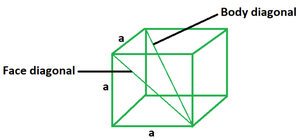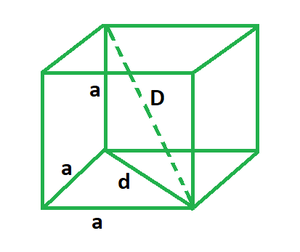# Diagonal of a Cube Formula

• Last Updated : 15 May, 2022

A cube is defined as a 3D solid object which has six square faces and all sides equal to each other. It is one of the five platonic solids and is also known as a regular hexahedron. Six square faces, eight vertices, and twelve edges make up the cube. Its length, breadth and height all are same as it is a square with all sides of the same length. The common line between any two faces of a cube is called its edge. It acts as a boundary line between two faces.Diagonal of a cube

A cube’s diagonal is the line segment connecting any two non-adjacent vertices of the cube. There are two types of cube diagonals, that is, face diagonal and body diagonal. A face diagonal joins two non-adjacent vertices via a single face while a body diagonal does the same by passing through the body of the cube. There are 12 face diagonals and 4 body diagonals possible inside a cube.

Face diagonal formula

Length of face diagonal of cube = √2a

where,

a is the side length of cube.

Body diagonal formula

Length of body diagonal of cube = √3a

where,

a is the side length of cube.

### DerivationSuppose we have a cube with side length a, face diagonal d and body diagonal D.

Now it is known that face diagonal is the hypotenuse of the right triangle it forms with two side lengths.

So, using Pythagoras theorem we get,

d2 = a2 + a2

d2 = 2a2

d = √2a   …….. (1)

Also, the face diagonal and one side length form a right triangle with body diagonal as hypotenuse.

Again using Pythagoras theorem we get,

D2 = a2 + d2

Using (1) we get,

D2 = a2 + (√2a)2

D2 = a2 + 2a2

D2 = 3a2

D = √3a

This derives the formulas for face diagonals and body diagonals of a cube.

### Sample Problems

Problem 1. Calculate the face diagonal of a cube of side length 2 m.

Solution:

We have,

a = 2

Using the formula we get,

d = √2a

= √2 (2)

= (1.414) (2)

= 2.83 m

Problem 2. Calculate the face diagonal of a cube of side length 5 m.

Solution:

We have,

a = 5

Using the formula we get,

d = √2a

= √2 (5)

= (1.414) (5)

= 7.07 m

Problem 3. Calculate the body diagonal of a cube of side length 3 m.

Solution:

We have,

a = 3

Using the formula we get,

D = √3a

= √3 (3)

= (1.732) (3)

= 5.196 m

Problem 4. Calculate the body diagonal of a cube of side length 7 m.

Solution:

We have,

a = 7

Using the formula we get,

D = √3a

= √3 (7)

= (1.732) (7)

= 12.12 m

Problem 5. Calculate the side length if the face diagonal of a cube is 6 m.

Solution:

We have,

d = 6

Using the formula we get,

d = √2a

=> a = d/√2

= 6/√2

= 4.24 m

Problem 6. Calculate the side length if the body diagonal of a cube is 13√3 m.

Solution:

We have,

D = 13√3

Using the formula we get,

D = √3a

=> a = D/√3

= 13√3/√3

= 13 m

Problem 7. Calculate the body diagonal of a cube if its face diagonal is 9√2 m.

Solution:

We have,

d = 9√2

Find the side length.

d = √2a

=> a = d/√2

= 9√2/√2

= 9 m

Now using the formula we get,

D = √3a

= √3 (9)

= 15.58 m

My Personal Notes arrow_drop_up